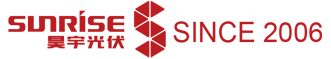﻿ What Are the Performance Parameters of Solar Panels? - Sunrise Energy Co., Ltd.• en

# What Are the Performance Parameters of Solar Panels?

Solar panel parameters are provided by the manufacturer under STC (standard test conditions). Under STC, the corresponding solar radiation is equal to 1000W/m2, the cell operating temperature is 25℃, and Am is 1.5. The parameters of solar cells are as follows:

## Solar panel ISC, short-circuit current

The short-circuit current is the maximum current generated by the solar panel, and its unit is ampere (A) or milliampere (mA). The value of short-circuit depends on the solar panel area, the solar radiation falling on the solar panel, and the battery technology.

Sometimes the manufacturer provides current density instead of current value. Current density is represented by "J", and short-circuit current density is represented by "JSC". Their relationship can be expressed by the following formula: JSC = ISC / A.

For example, if a solar panel has a current density of 50mA/㎠ and an area of 300 ㎠ at STC, the short-circuit current can be determined as follows: ISC=JSC * area = 50 * 300 = 15000mA=15A.

## Solar panel VOC, open-circuit voltage

The open-circuit voltage is the maximum voltage that the solar panel can generate under open-circuit conditions. Its measurement unit is volt (V) or millivolt (mV). The value of VOC depends on the solar panel technology and operating temperature.

## Solar panel PM, peak power

Measurement unit is usually W. The maximum power represents the maximum power that the solar panel can generate under STC. It is measured in Wpeak or simply WP.

In addition to STC, the solar panel also has PM at different levels of radiation and operating temperatures. The solar panel can work under different combinations of current and voltage. But it can only produce the maximum power PM at a specific combination of voltage and current, which can be expressed by the following formula: PM = IM×VM.

## Solar panel Imp, current at maximum power point

It represents the current generated by the solar panel when it works at the maximum power point. Its value is always less than the short-circuit current (ISC). The measurement unit is ampere (A) or milliampere (mA).

## Solar panel Vmp, voltage at maximum power point

It represents the voltage generated by the solar panel when it works at the maximum power point. Its measurement units are volt (V) or millivolt (mV).

## Solar panel FF (%), fill factor

It is expressed as a percentage (%), and the higher the fill factor, the better the battery. The fill factor is defined as the maximum power (at MPP) divided by the product of ISC and VOC. We can see that the fill factor is always less than 1.

Due to the difficulty in measuring the conversion efficiency quickly, the fill factor is often measured instead. It is usually expressed by the following formula: FF = Pm / (Isc×Voc), efficiency.

The efficiency of the solar panel is defined as the maximum output power (PM) divided by the input power (PIN). It is measured in percentage (%), indicating the percentage of input solar power converted into electric energy. The input power is power density. Efficiency can be calculated as follows: = PM / (PIN×Area).

•